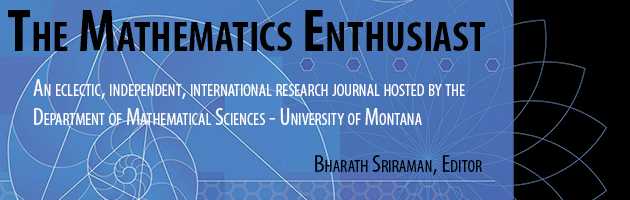•
•6

1-2

#### Abstract

We discuss the significance of making connections between the verbal, algebraic, and geometric representations of basic mathematical objects for students’ understanding of mathematical instructions. Our survey of 499 students enrolled in a precalculus university course reveals that such connections are not always present, even if the objects themselves are familiar to the students. We stress that the ability of making these connections needs to be specifically addressed in teaching mathematics at various levels. A proper attention to the matter contributes to the formation of students’ mathematical background, which makes a difference for their success in study of calculus, in particular.

213

238

COinS